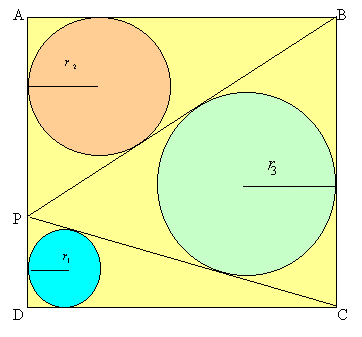#### You may also like### Baby Circle

A small circle fits between two touching circles so that all three circles touch each other and have a common tangent? What is the exact radius of the smallest circle?A circle is inscribed in an equilateral triangle. Smaller circles touch it and the sides of the triangle, the process continuing indefinitely. What is the sum of the areas of all the circles?### Kissing

Two perpendicular lines are tangential to two identical circles that touch. What is the largest circle that can be placed in between the two lines and the two circles and how would you construct it?

# Sangaku

##### Age 16 to 18 Challenge Level:

The square $ABCD$ has sides of length 1 unit and it is split into three triangles by the lines $BP$ and $CP$. If $P$ is the midpoint of $AD$, find the radii of the inscribed circles of these triangles.

Now suppose the lengths $AP$ and $PD$ are $(1- p)$ and $p$ respectively. Find the radii of the three circles $r_1$, $r_2$and $r_3$ in terms of $p$ and plot, on the same axes, the graphs of $r_1$, $r_2$ and $r_3$ as $p$ varies from 0 to 1. Can the ratio of the radii $r_1 : r_2 : r_3$ ever take the value $1:2:3$?Notes and Background

Sangaku in Japanese means a mathematics tablet. During the Edo period (1603-1867) when Japan was cut off from the western world, people of all classes produced theorems in Euclidean geometry as beautifully coloured drawings on wooden tablets to be hung in a temple. Proofs were rarely given. The tablets challenged other geometers: "See if you can prove this."